Question

# 1. Ceres is the largest asteroid. Its mean radius is 476 km and its mass is...

1. Ceres is the largest asteroid. Its mean radius is 476 km and its mass is 9.4×10^20 kg. Using this, and G=6.67×10^−11 Nm^ 2 kg^−2, and approximating it as a sphere, compute the speed required to escape the gravity on the surface of Ceres.

Speed= ___ m.s^−1. (to two significant figures, don't use scientific notation)

2.  Consider the mechanical energy of a body in geostationary orbit above the Earth's equator, at rGS​ = 42000 km.

Consider the mechanical energy of the same body on Earth at the South pole, at re​=6400 km. For this problem, we consider the Earth to be spherical. (Remember, the object at the equator is in orbit, the object at the Pole is not in orbit.)

G = 6.67×10^−11 Nm^2 kg^−2, and the mass of the Earth is M = 5.97×10^24 kg

What is the difference in the mechanical energy per kilogram between the two?

E= ___ MJ.kg^−1 (to two significant figures, don't use scientific notation)

3. Consider the mechanical energy of a body at rest on the ground at the Earth's equator, at re​=6400 km

Consider the mechanical energy of the same body at rest on the ground at the South pole, at re​=6400 km. For this problem, we consider the Earth to be spherical.

(Remember, the object at the equator traces a circular path, the object at the Pole does not.)

G=6.67×10^−11 Nm^2 kg^−2, and the mass of the Earth is M = 5.97×10^24 kg

1. How much more mechanical energy per kilogram does an object on the ground at the Equator than on the ground at the Pole?
2. E= ___ MJ.kg^−1 . (2 sig figs, do not use scientific notation)

1) escape speed = sqrt(2GM/R)

where G = gravitational constant

M = mass , R = radius

V(esc) = sqrt(2*6.67*10^-11*9.4*10^20 / 476000^2)

= 0.55 m/s

so the escape speed is 0.55 m/s

#### Earn Coins

Coins can be redeemed for fabulous gifts.

Similar Homework Help Questions
• ### Question 1 of 10 > Attempt 4 Consider a 495 kg satellite in a circular orbit...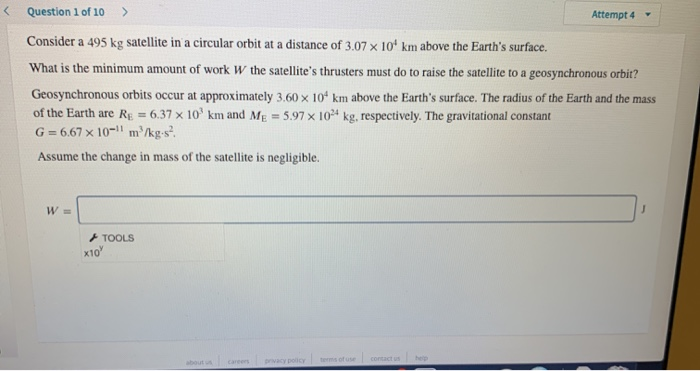Question 1 of 10 > Attempt 4 Consider a 495 kg satellite in a circular orbit at a distance of 3.07 x 10 km above the Earth's surface. What is the minimum amount of work W the satellite's thrusters must do to raise the satellite to a geosynchronous orbit? Geosynchronous orbits occur at approximately 3.60 x 10 km above the Earth's surface. The radius of the Earth and the mass of the Earth are Re = 6,37 x 10 km...

• ### An asteroid with mass of 2.59 1020 kilograms (or 4.3410-5 MEarth) is kept in its orbit...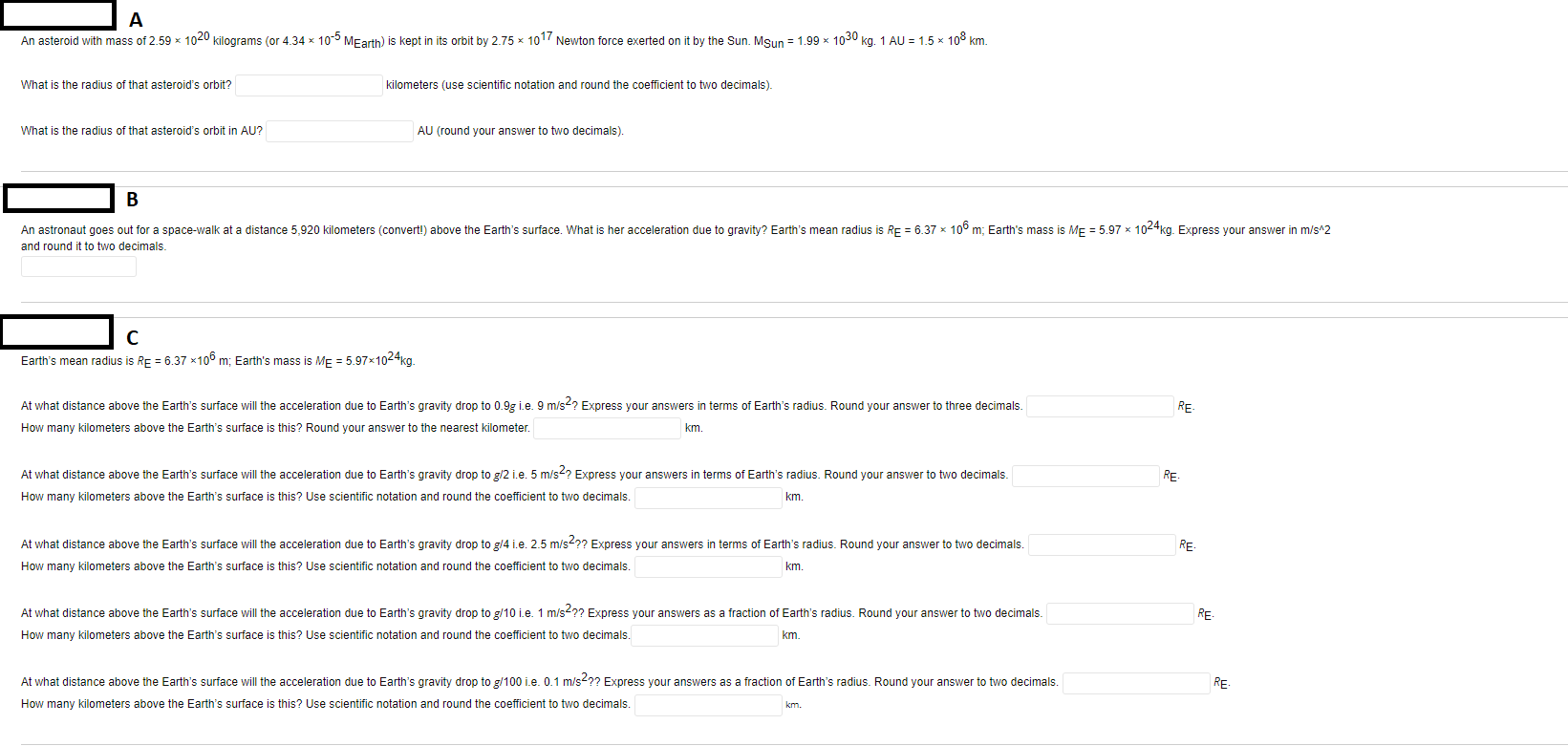An asteroid with mass of 2.59 1020 kilograms (or 4.3410-5 MEarth) is kept in its orbit by 2.75 * 1017 Newton force exerted on it by the Sun. Msun = 1.99 % 1030 kg. 1 AU = 1.5 * 108 km. What is the radius of that asteroid's orbit? kilometers (use scientific notation and round the coefficient to two decimals). What is the radius of that asteroid's orbit in AU? AU (round your answer to two decimals). An astronaut goes...

• ### Consider a 455 kg satellite in a circular orbit at a distance of 3.06 x 10...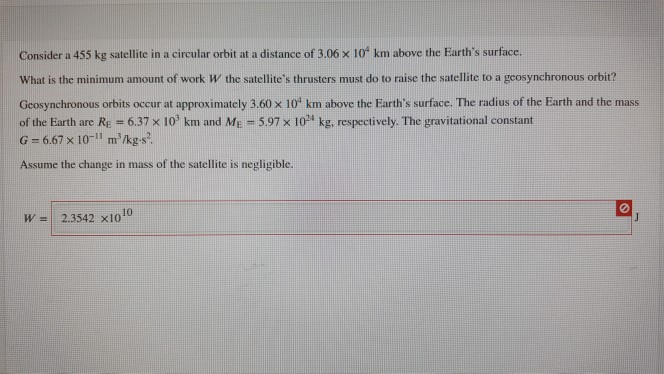Consider a 455 kg satellite in a circular orbit at a distance of 3.06 x 10 km above the Earth's surface. What is the minimum amount of work W the satellite's thrusters must do to raise the satellite to a geosynchronous orbit? Geosynchronous orbits occur at approximately 3.60 X 10 km above the Earth's surface. The radius of the Earth and the mass of the Earth are Re = 6,37 x 10 km and Me = 5.97 x 10 kg,...

• ### Question 1 of 10 > Attempt 2 - Consider a 455 kg satellite in a circular orbit at a distance of 3.06 x 10 km abov...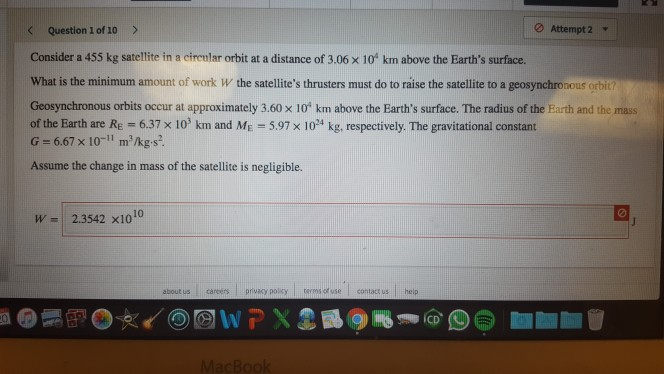Question 1 of 10 > Attempt 2 - Consider a 455 kg satellite in a circular orbit at a distance of 3.06 x 10 km above the Earth's surface. What is the minimum amount of work W the satellite's thrusters must do to raise the satellite to a geosynchronous orbit Geosynchronous orbits occur at approximately 3.60 x 10 km above the Earth's surface. The radius of the Earth and the mass of the Earth are Rę = 6.37 x 10...

• ### Consider a 455 kg satellite in a circular orbit at a distance of 3.02 x 104...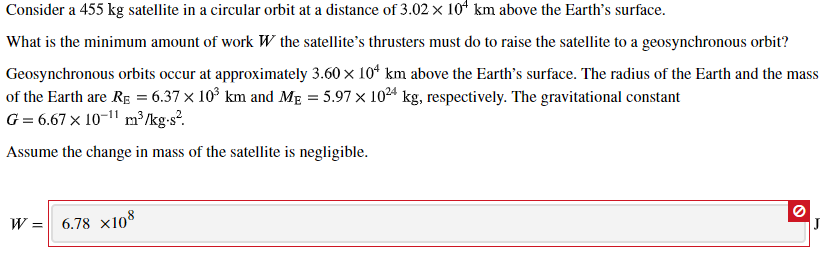Consider a 455 kg satellite in a circular orbit at a distance of 3.02 x 104 km above the Earth's surface. What is the minimum amount of work W the satellite's thrusters must do to raise the satellite to a geosynchronous orbit? Geosynchronous orbits occur at approximately 3.60 x 104 km above the Earth's surface. The radius of the Earth and the mass of the Earth are Re = 6.37 % 10% km and Me = 5.97 x 1024 kg,...

• ### Consider a 475 kg satellite in a circular orbit at a distance of 3.06 x 104...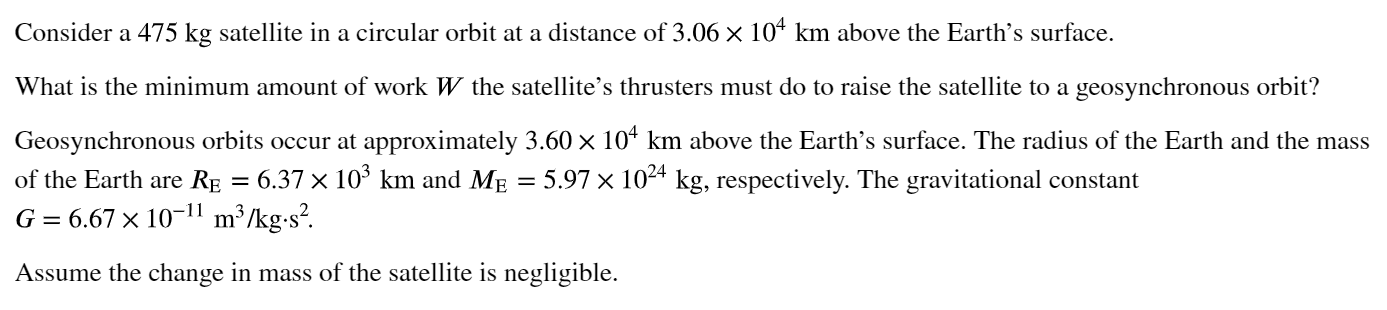Consider a 475 kg satellite in a circular orbit at a distance of 3.06 x 104 km above the Earth's surface. What is the minimum amount of work W the satellite's thrusters must do to raise the satellite to a geosynchronous orbit? Geosynchronous orbits occur at approximately 3.60 x 104 km above the Earth's surface. The radius of the Earth and the mass of the Earth are RE = 6.37 x 109 km and Me = 5.97 x 1024 kg,...

• ### Consider a 495 kg satellite in a circular orbit at a distance of 3.02 x 104...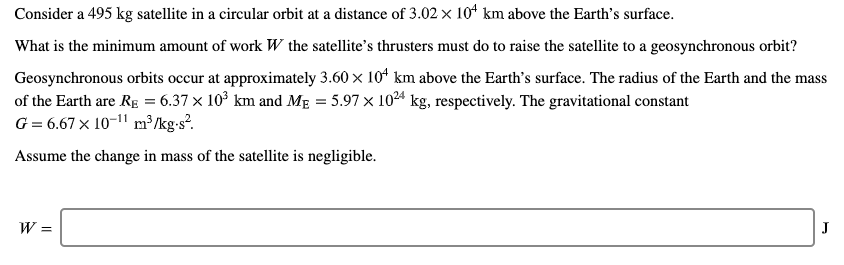Consider a 495 kg satellite in a circular orbit at a distance of 3.02 x 104 km above the Earth's surface. What is the minimum amount of work W the satellite's thrusters must do to raise the satellite to a geosynchronous orbit? Geosynchronous orbits occur at approximately 3.60 x 104 km above the Earth's surface. The radius of the Earth and the mass of the Earth are RE = 6.37 x 103 km and Me = 5.97 x 1024 kg,...

• ### Consider a 455 kg satellite in a circular orbit at a distance of 3.02 x 104...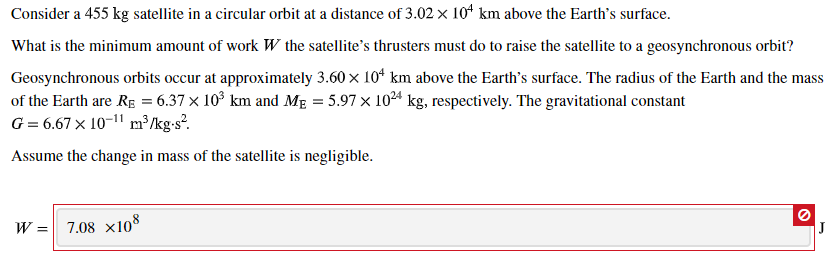Consider a 455 kg satellite in a circular orbit at a distance of 3.02 x 104 km above the Earth's surface. What is the minimum amount of work W the satellite's thrusters must do to raise the satellite to a geosynchronous orbit? Geosynchronous orbits occur at approximately 3.60 x 107 km above the Earth's surface. The radius of the Earth and the mass of the Earth are RE = 6.37 x 109 km and Me = 5.97 x 1024 kg,...

• ### Consider a spherical asteroid with a radius of 20 km and a mass of 9.25 ✕...

Consider a spherical asteroid with a radius of 20 km and a mass of 9.25 ✕ 1015 kg. Assume the asteroid is roughly spherical. (a) What is the acceleration due to gravity on the surface of this asteroid? m/s2 (b) Suppose the asteroid spins about an axis through its center, like the Earth, with a rotational period T. What is the smallest value T can have before loose rocks on the asteroid's equator begin to fly off the surface? h

• ### Newton's constant of gravitation G is 6.67×10-11 in the system of units we use with mass...

Newton's constant of gravitation G is 6.67×10-11 in the system of units we use with mass in kilograms, length in meters, and time in seconds. The radius of Earth is approximately 6.378 ×106 m, and its mass is 5.97 × 1024 kg. With all this you can evaluate conservation of energy from Earth's surface and find an escape velocity of about 11.2 km/s from Now suppose we wanted to orbit a spacecraft in low Earth orbit just a few hundred...